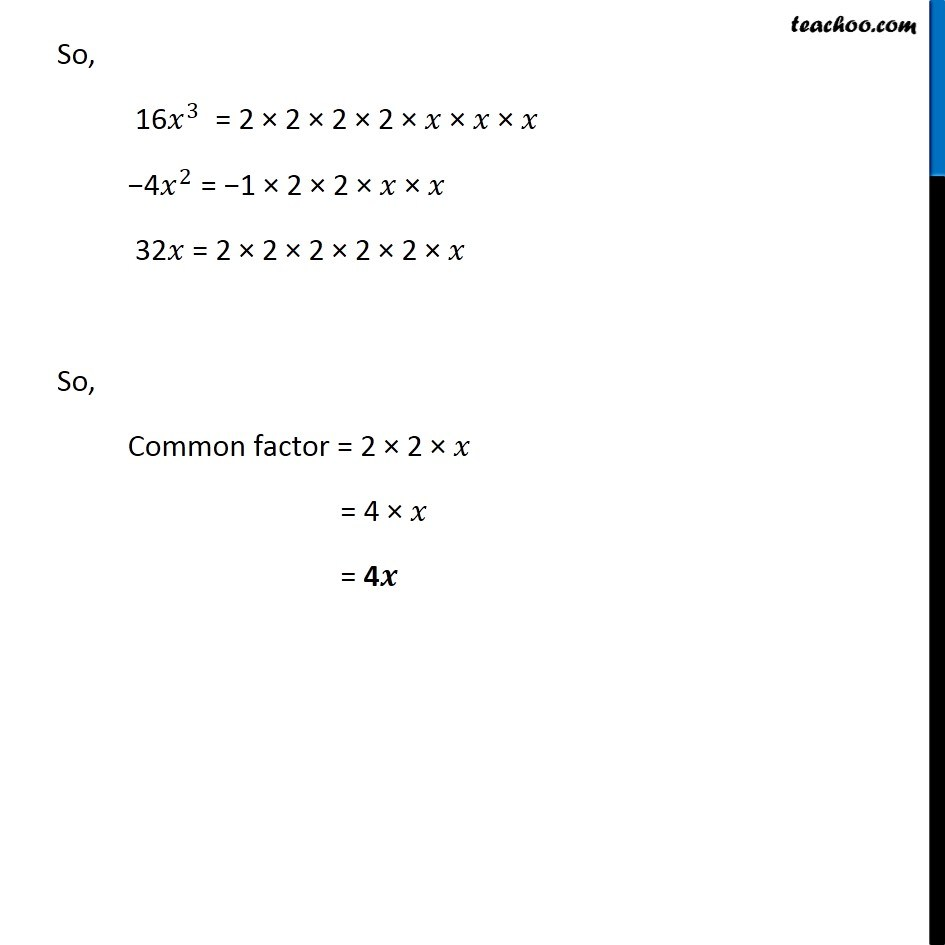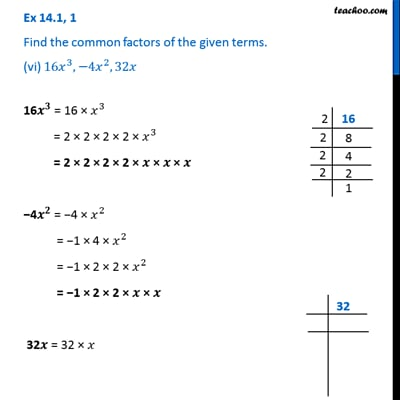Ex 14.1

Chapter 14 Class 8 Factorisation
Serial order wiseThis video is only available for Teachoo black users

Get live Maths 1-on-1 Classs - Class 6 to 12

### Transcript

Ex 14.1, 1 Find the common factors of the given terms. (vi) 〖16𝑥〗^3,−〖4𝑥〗^2, 32𝑥 16𝑥^3 = 16 × 𝑥^3 = 2 × 2 × 2 × 2 × 𝑥^3 = 2 × 2 × 2 × 2 × 𝑥 × 𝑥 × 𝑥 −4𝑥^2 = −4 × 𝑥^2 = −1 × 4 × 𝑥^2 = −1 × 2 × 2 × 𝑥^2 = −1 × 2 × 2 × 𝑥 × 𝑥 32𝑥 = 32 × 𝑥 = 2 × 2 × 2 × 2 × 2 × 𝑥 So, 16𝑥^3 = 2 × 2 × 2 × 2 × 𝑥 × 𝑥 × 𝑥 −4𝑥^2 = −1 × 2 × 2 × 𝑥 × 𝑥 32𝑥 = 2 × 2 × 2 × 2 × 2 × 𝑥 So, Common factor = 2 × 2 × 𝑥 = 4 × 𝑥 = 4𝒙